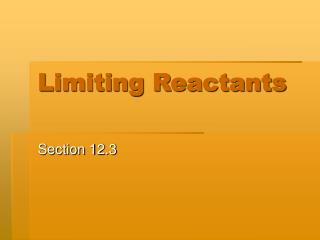DownloadDownload PresentationLimiting Reactants

# Limiting Reactants

Download Presentation## Limiting Reactants

- - - - - - - - - - - - - - - - - - - - - - - - - - - E N D - - - - - - - - - - - - - - - - - - - - - - - - - - -
##### Presentation Transcript

1. Limiting Reactants Section 12.3

2. Limiting Reactants Rarely are the reactants in a chemical equation present in the exact ratios specified by the balanced equation. Generally, there is too much of one and not enough of the other. The chemical reaction will stop once one of the reactants is used up. This leaves the remainder of the reactant that was in excess unreacted. The chemical that you ran out of was the limiting reactant.

3. Limiting Factor Notesheet Consider the following recipe: 3 cups flour + 2 eggs + 1 cup sugar + 2 tsp baking powder  12 muffins A quick check of the pantry shows that the following quantities are available. How many muffins could be made with respect to each ingredient? 9 cups of flour could make ___36___ muffins 4 eggs could make __24__ muffins 8 cups of sugar could make __96___ muffins 10 tsp baking powder could make __60___ muffins

4. Limiting Factor Note sheet 3 cups flour + 2 eggs + 1 cup sugar + 2 tsp baking powder  12 muffins What is the maximum number of muffins that could be made considering ALL ingredients? __24___ Which ingredient would you run out of first? _eggs____ This ingredient would be referred to as the __limiting_ __reactant____. The other ingredients are said to be __in __ excess . When you run out of an ingredient, you stop producing the _product__.

5. Limiting Factor Note sheetcont’d… Consider the following “recipe” (equation): 2 mol of Al + 3 mol of Cl2 2 mol of AlCl3 A quick check of the chemistry lab shows that the following quantities are available. How much product could be made from each? (How many times could you make the recipe?) 10 moles Al ______ 12 moles Cl2 _____

6. What is the maximum amount of AlCl3 that could be made considering all the ingredients? ________ We would say the aluminum is _________________ and the chlorine is _________________. What if you were presented with grams of each reactant? Let’s say there are 100 g of Al and 125 g of Cl2 available. How much product could be formed? (Remember, the coefficients in the equation are mole numbers) 100 g of Al would yield ________ mol of product. 125 g of Cl2 would yield ________ mol of product. How much product could be made considering both reactants? ________ The limiting factor would be ________.

7. Calculating the Product when the Reactant is Limited In the following equation: If 200 g of sulfur reacts with 100 g of chlorine what mass of sulfur chloride will be produced? 200 100 x S8 + 4 Cl2 4S2Cl2 256 280 536 Sulfur Chlorine 200 g = x g 100 g = x g 256 g 536 g 280 g 536 g 256x = 107200 280x = 53600 x = 418.75 g x = 191.42 g  lowest #

8. Steps for Calculating Limiting Reactants • Balance the equation • Find the mass of each of the compounds (molar mass x’s coefficient) • Set up ratios for each of the reactants and solve • Look for the lowest number – this is your limiting reactant

9. Limiting Reactant Practice I Balance the following formula: N2 + H2 NH3 1. If you had 112 grams of nitrogen, what is the maximum number of grams of NH3 that could be produced? 2. If you had 18 grams of hydrogen, what is the maximum number of grams of NH3 that could be produced? 3. What is the maximum number of grams of NH3 that can be produced with 112 grams of nitrogen and 18 grams of hydrogen? 5. What is the limiting reactant?

10. To Determine How Much More of the Limiting Reactant is Needed to Use Up the Excess Reactant and Finish the Reaction: 200 100 S8 + 4 Cl2  4 S2Cl2 256 280 200 g = __x__ 256 g 280 g 256x = 56000 x = 218.75 g - 100 g = 118.75 g total needed already used additional needed to complete reaction x